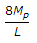# Civil Engineering - UPSC Civil Service Exam Questions

11.

A uniform simply supported beam is subjected to a clockwise moment at the left end. What is the moment required at the right end so that rotation of the right end is zero ?

 A. 2 m B. m C. m/2 D. m/3

Explanation:

No answer description available for this question. Let us discuss.

12.

A cylindrical bar of 20 mm diameter and 1 m length is subjected to a tensile test. Its longitudinal strain is 4 times that of its lateral strain. If the modulus of elasticity is 2 x 105 N/mm2, then its modulus of rigidity will be

 A. 8 x 106 N/mm2 B. 8 x 105 N/mm2 C. 0.8 x lO4 N/mm2 D. 0.8 x 105 N/mm2

Explanation:

No answer description available for this question. Let us discuss.

13.

Clapeyron's theorem is applied to

 A. simply supported beam B. fixed and continuous beam C. propped cantilever beam D. continuous beam only

Explanation:

No answer description available for this question. Let us discuss.

14.

A fill having a volume of 150, 000 cm is to be constructed at a void ratio of 0.8. The borrow pit soil has a void ratio of 1.4. The volume of soil required (in cubic metres) to be excavated from the borrow pit will be

 A. 1, 87, 500 B. 2, 00, 000 C. 2, 10, 000 D. 2, 50, 000

Explanation:

No answer description available for this question. Let us discuss.

15.

A fixed beam as shown in Fig. I is loaded with a U.D.L. over the entire span, the total load being W; when load was just increased to W1, the deformed shape as shown in Fig. II was seen. The value of W1 (plastic moment of resistance = Mp) is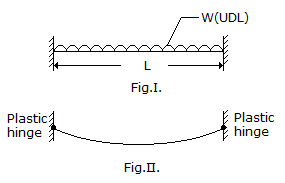A.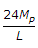B.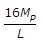C.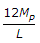D.